Скачать презентацию Wireless Communications Lecture — 3 Rayleigh Fading and

masters lect 4 week 2.pptx

• Количество слайдов: 25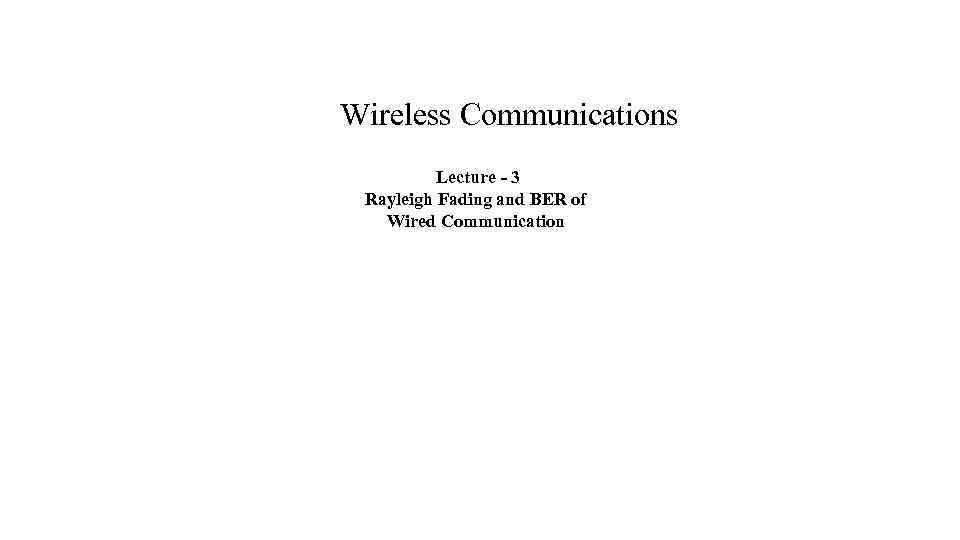Wireless Communications Lecture - 3 Rayleigh Fading and BER of Wired Communication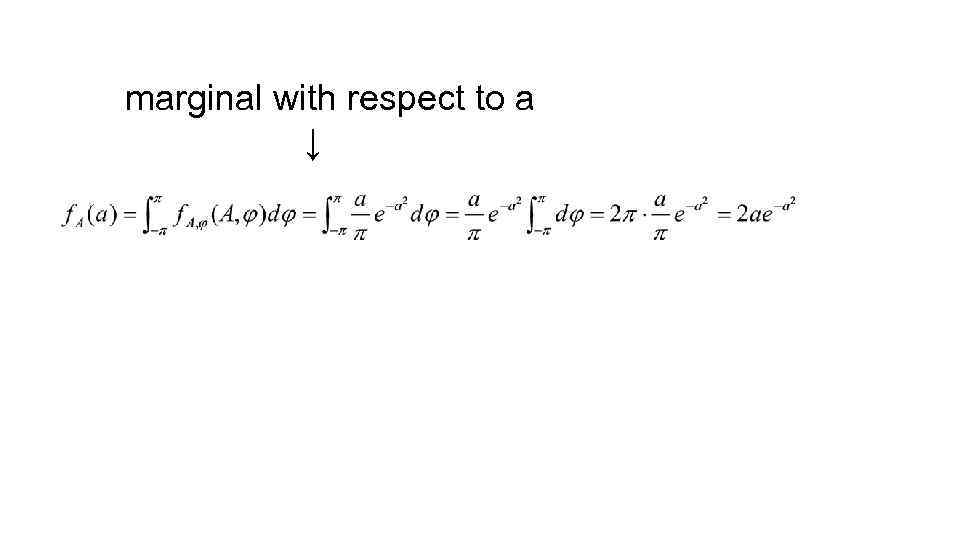marginal with respect to a ↓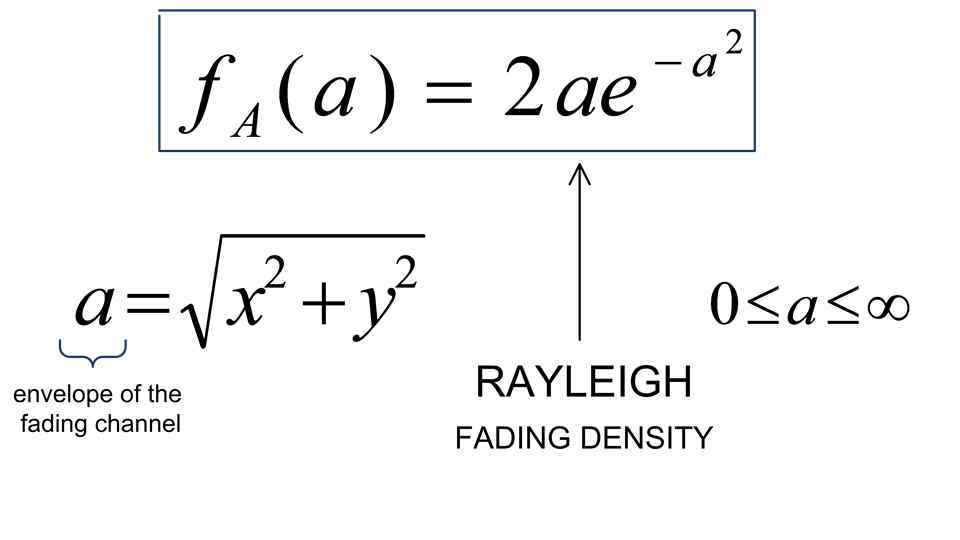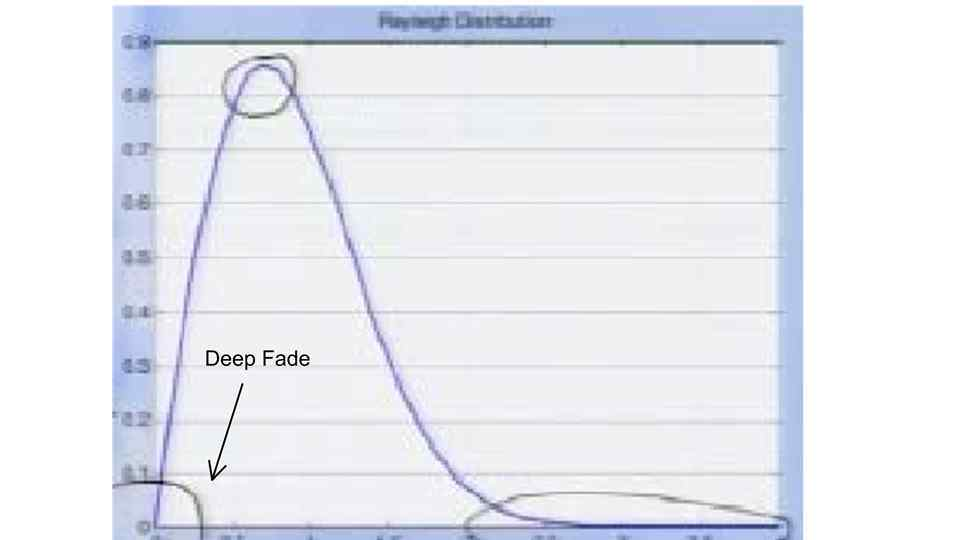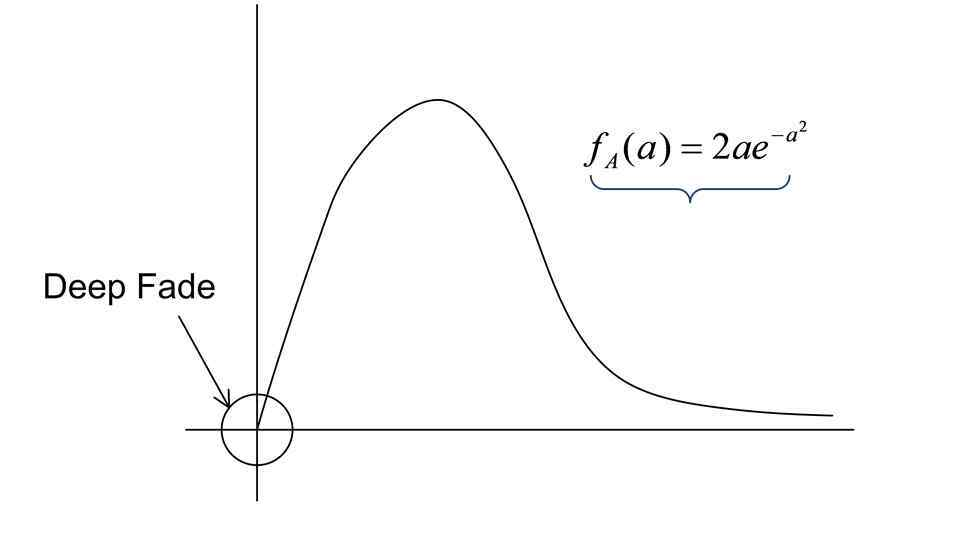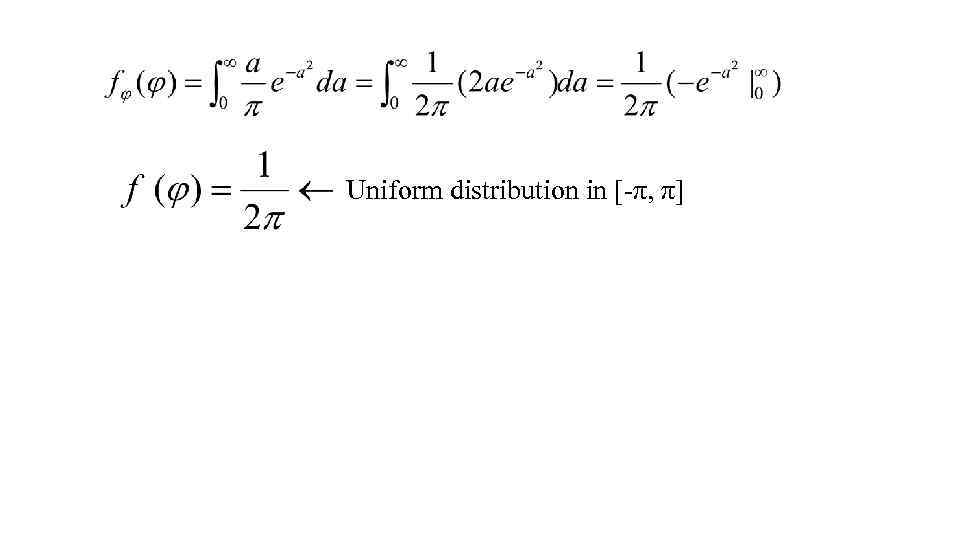Uniform distribution in [-π, π]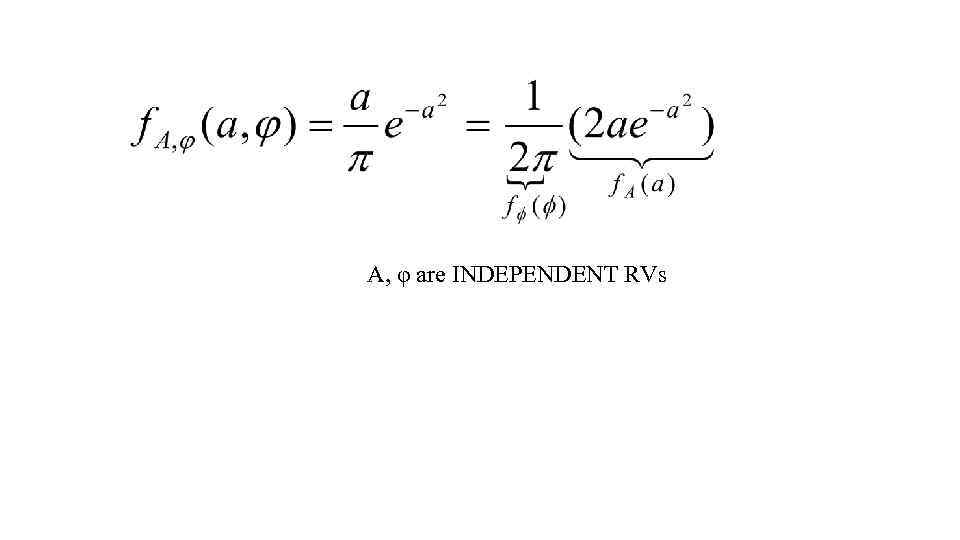A, φ are INDEPENDENT RVs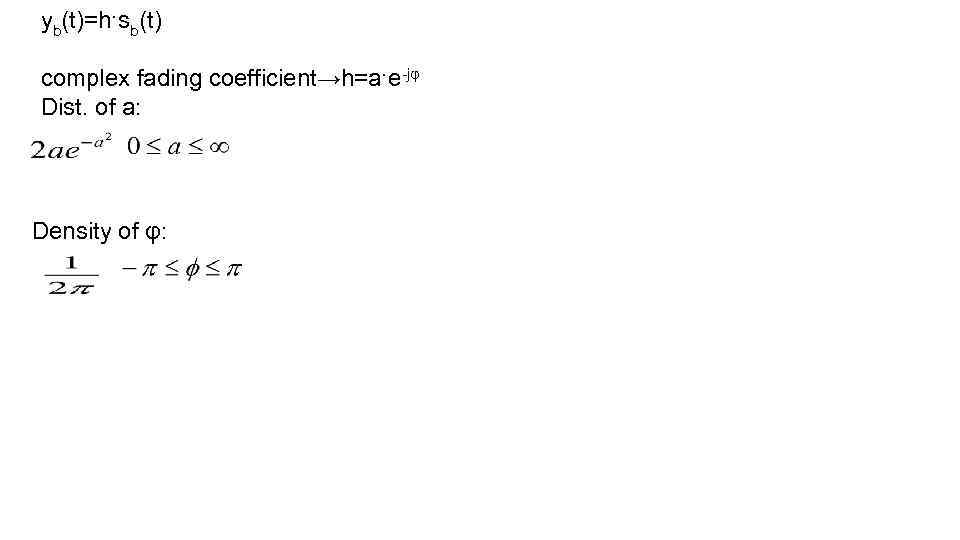yb(t)=h·sb(t) complex fading coefficient→h=a·e-jφ Dist. of a: Density of φ: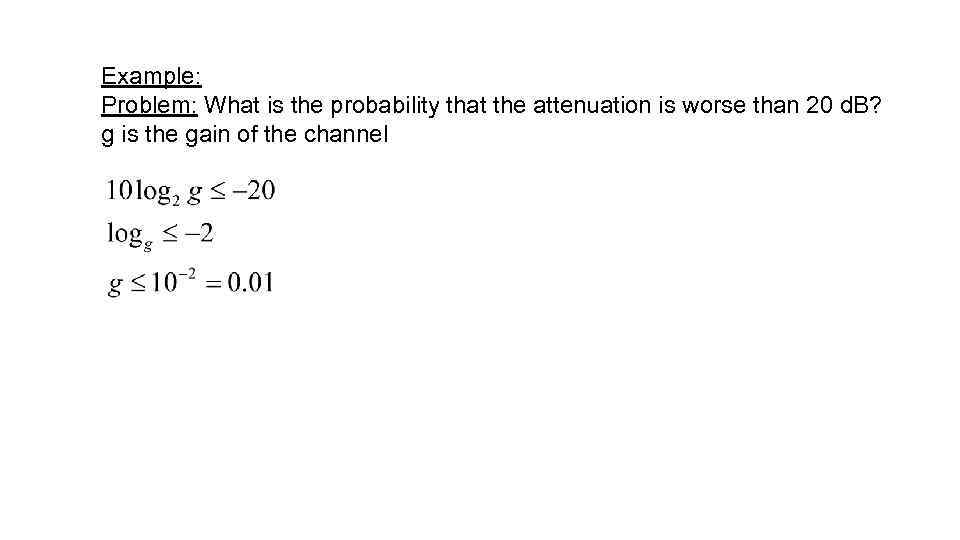Example: Problem: What is the probability that the attenuation is worse than 20 d. B? g is the gain of the channel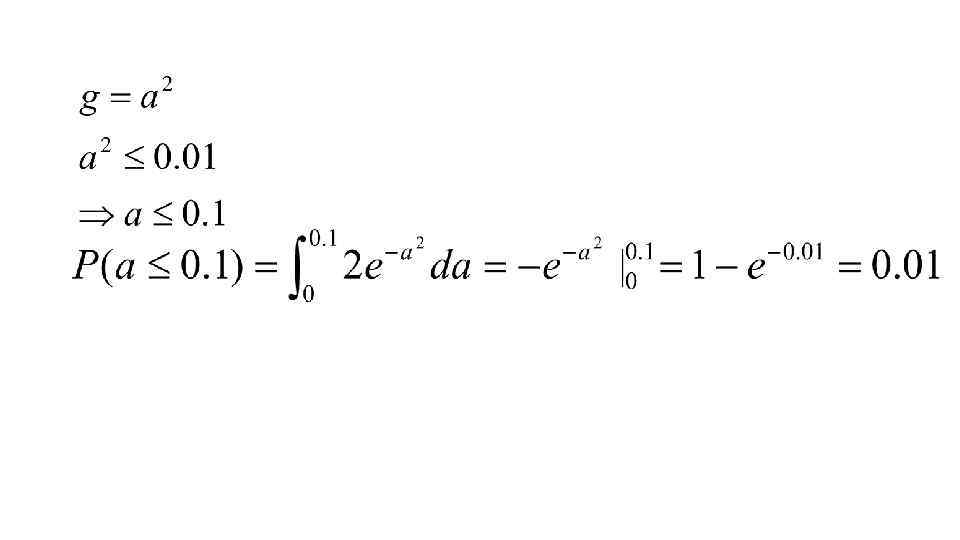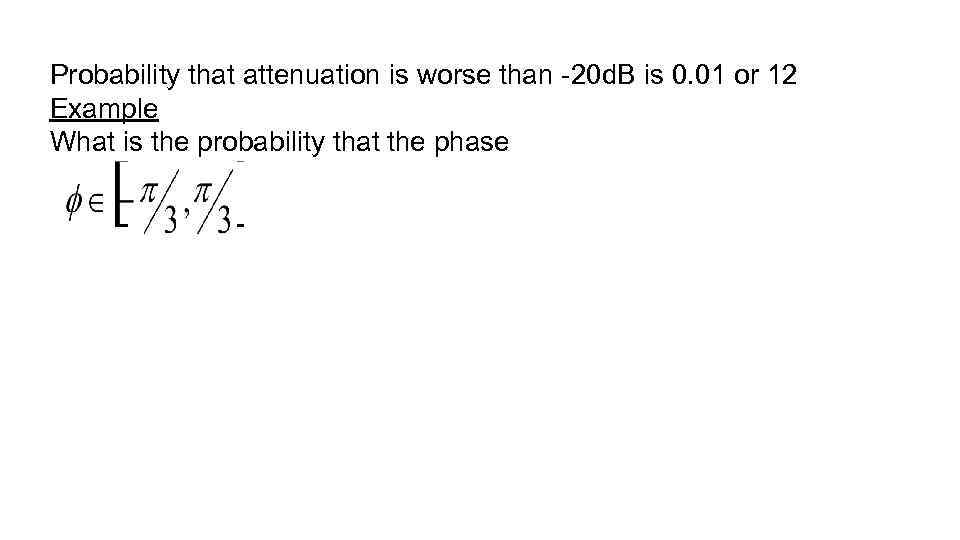Probability that attenuation is worse than -20 d. B is 0. 01 or 12 Example What is the probability that the phase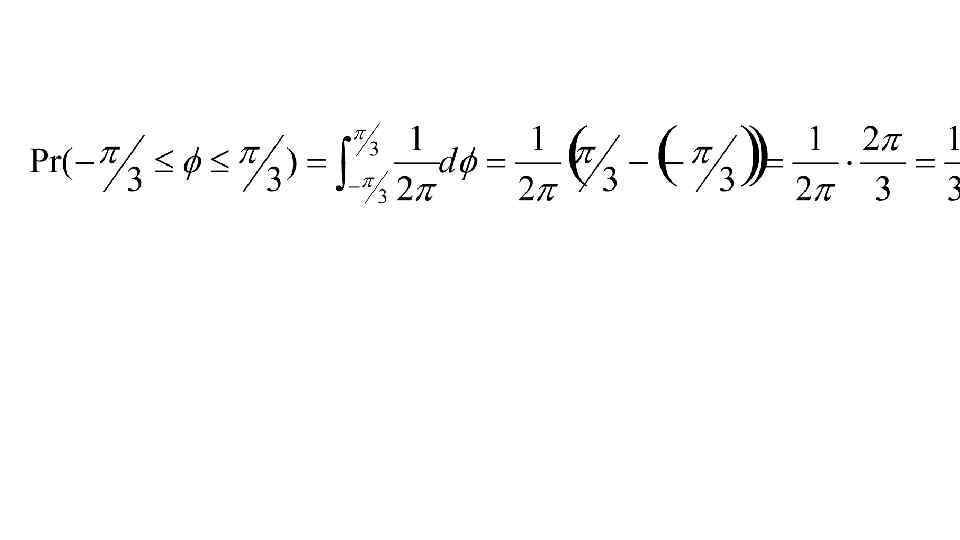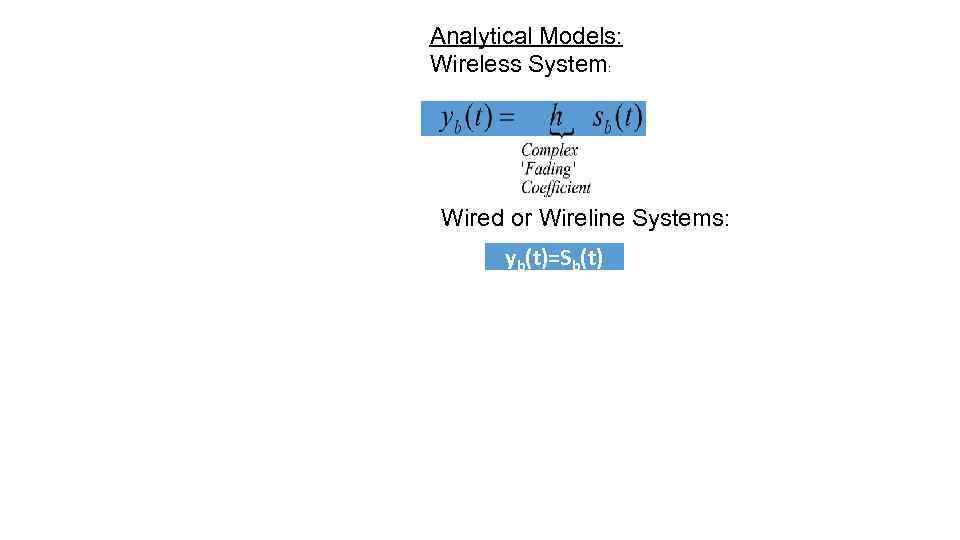Analytical Models: Wireless System: Wired or Wireline Systems: yb(t)=Sb(t)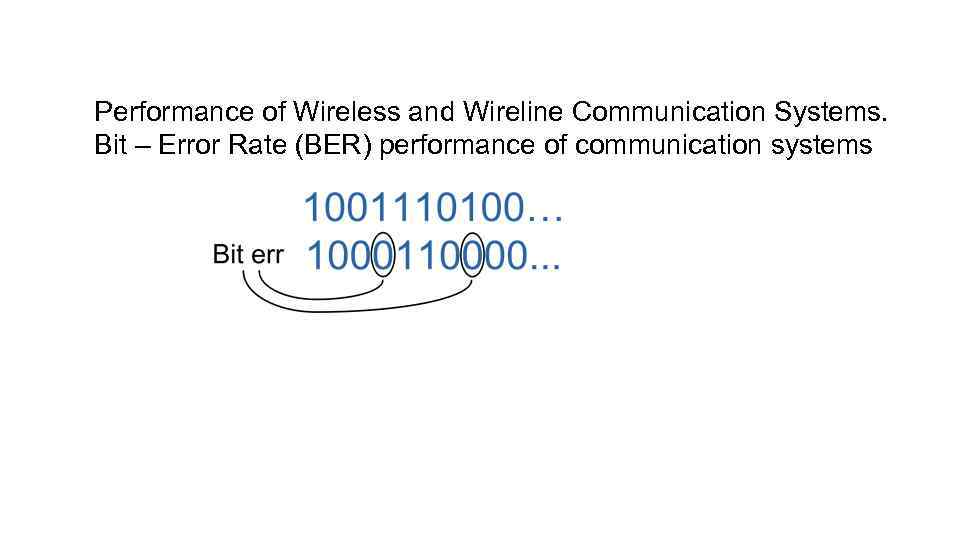Performance of Wireless and Wireline Communication Systems. Bit – Error Rate (BER) performance of communication systems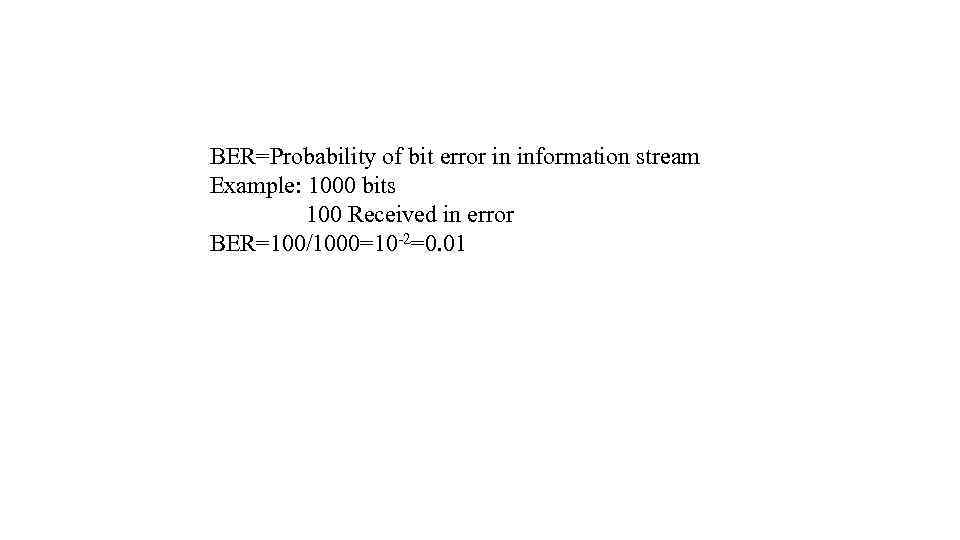BER=Probability of bit error in information stream Example: 1000 bits 100 Received in error BER=100/1000=10 -2=0. 01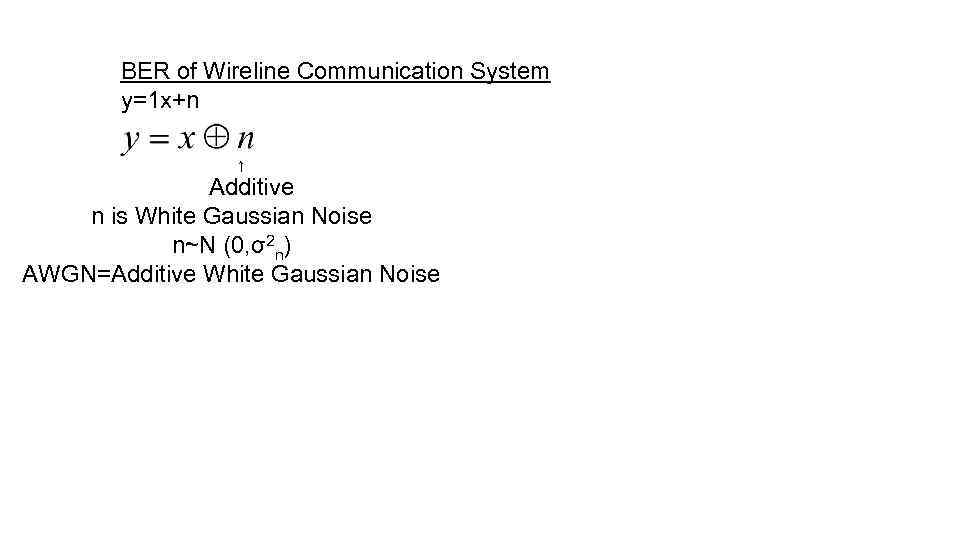BER of Wireline Communication System y=1 x+n ↑ Additive n is White Gaussian Noise n~N (0, σ2 n) AWGN=Additive White Gaussian Noise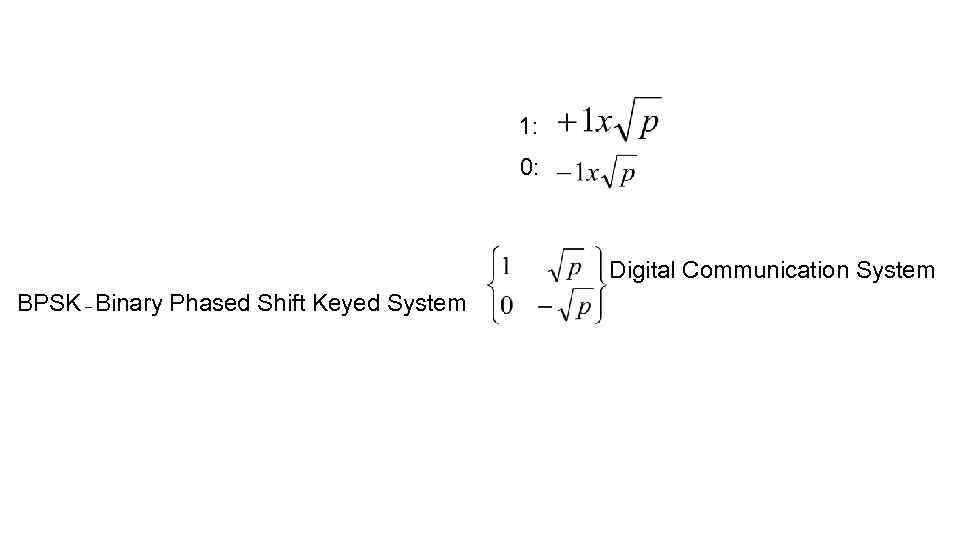1: 0: Digital Communication System BPSK – Binary Phased Shift Keyed System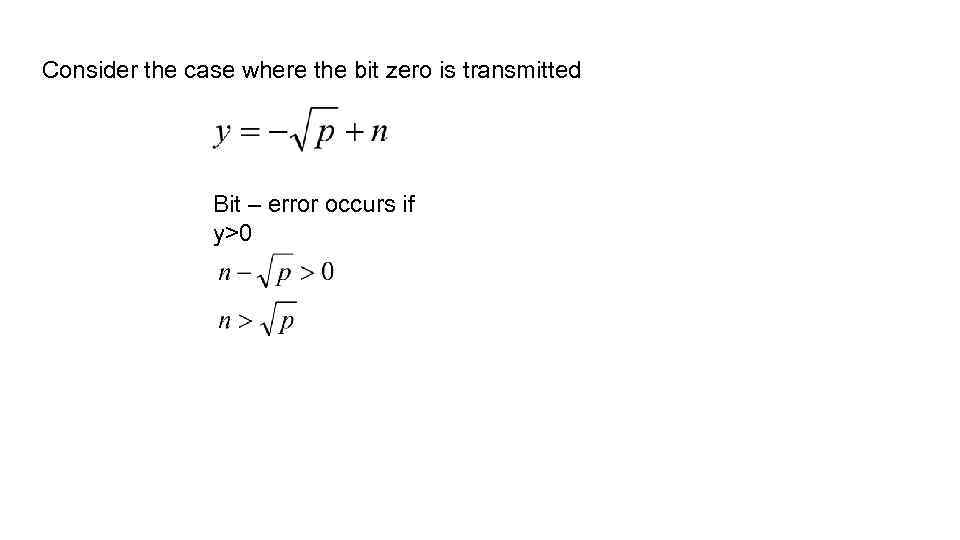Consider the case where the bit zero is transmitted Bit – error occurs if y>0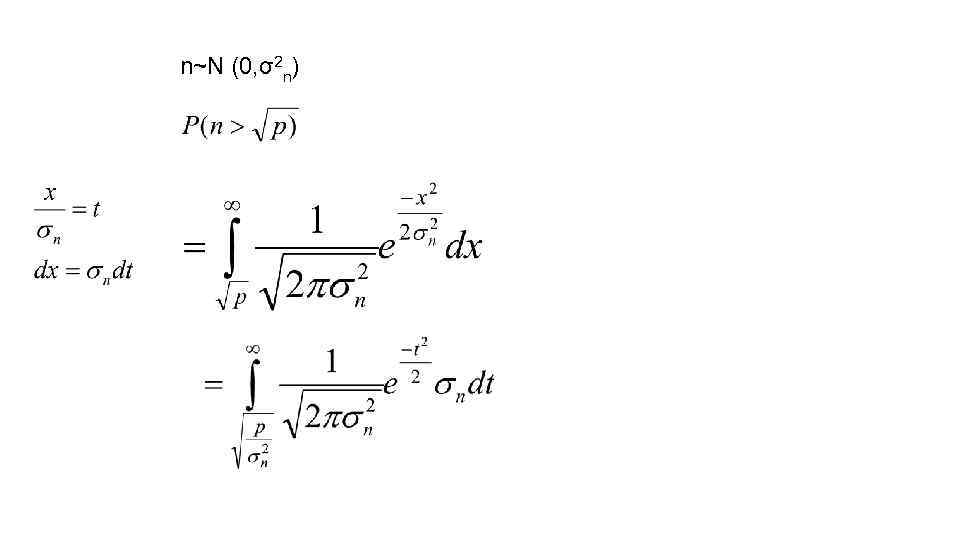n~N (0, σ2 n)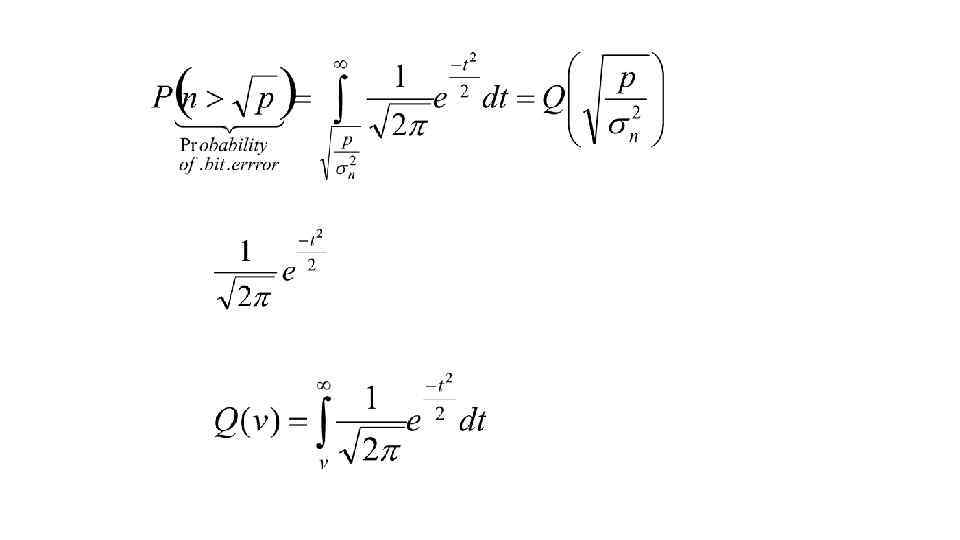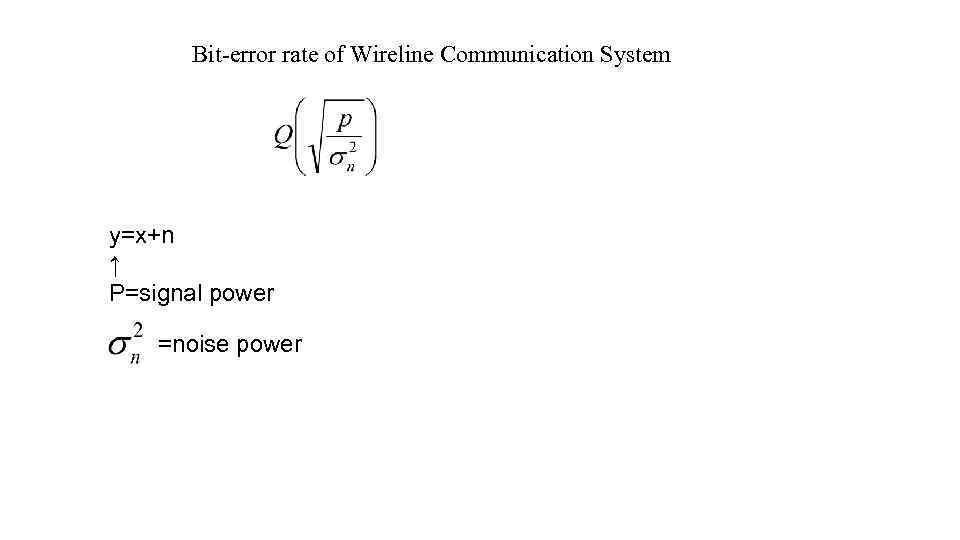Bit-error rate of Wireline Communication System y=x+n ↑ P=signal power =noise power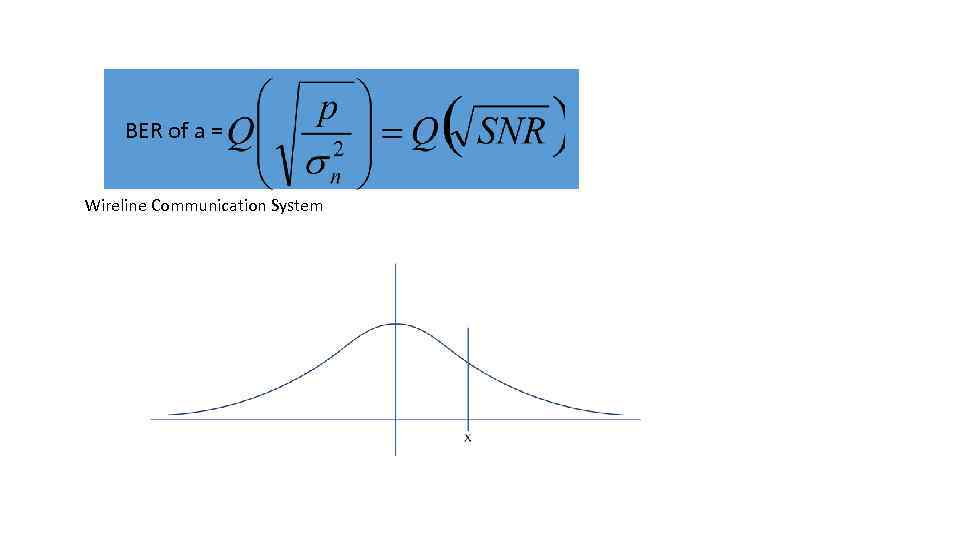BER of a = Wireline Communication System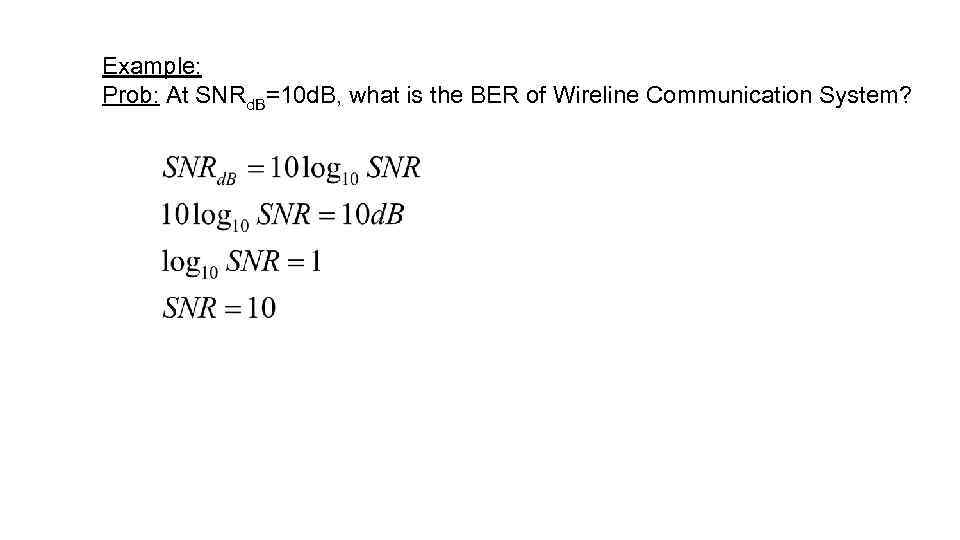Example: Prob: At SNRd. B=10 d. B, what is the BER of Wireline Communication System?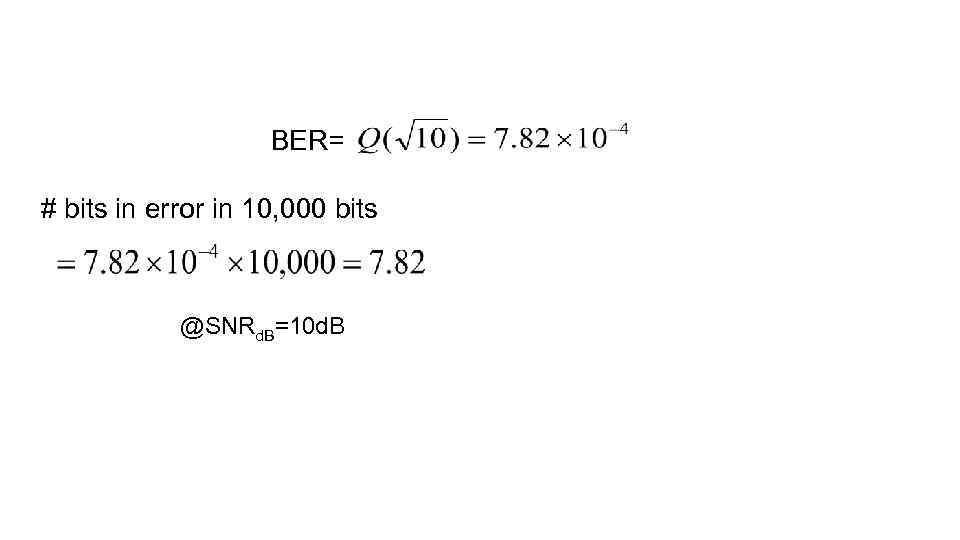BER= # bits in error in 10, 000 bits @SNRd. B=10 d. B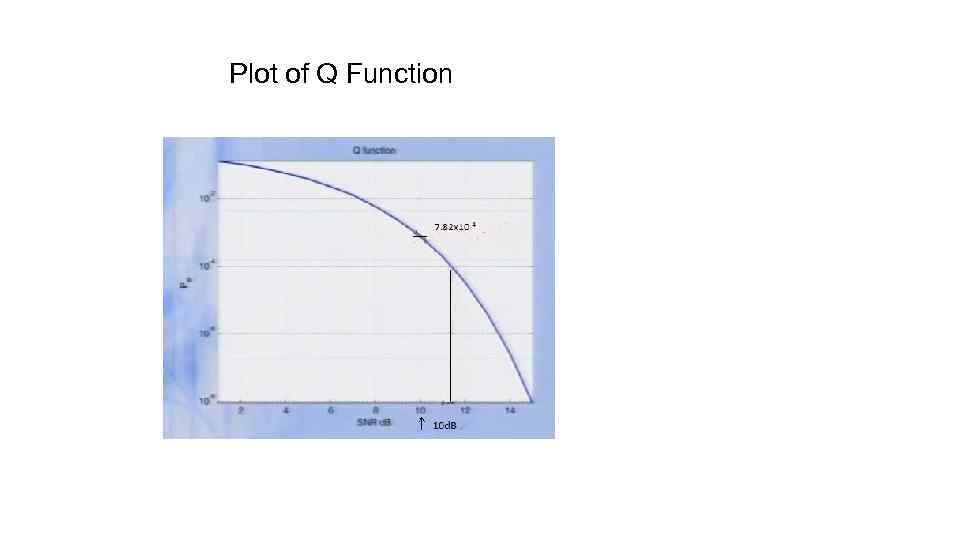Plot of Q Function